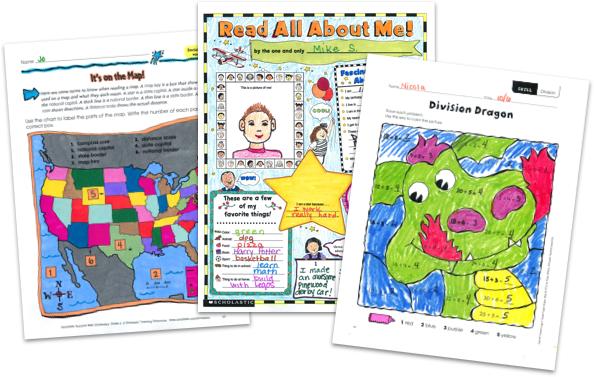MY FILE CABINET# 5th Grade Math Worksheets From Scholastic TeachablesScholastic Teachables (formerly Scholastic Printables) has more than 1,200 5th grade math worksheets that span many different fifth grade math skills! Sign up today and get instant online access to 5th grade math worksheets, lesson plans, practice pages, and more to cover all essential math skills. Plus, you'll get access to more than 30,000 award-winning printables and leveled learning collections in reading and math to reach every learner.

Scholastic Teachables (formerly Scholastic Printables) has more than 1,200 5th grade math worksheets that span many different fifth grade math skills! Sign up today and get instant online access to 5th grade math worksheets, lesson plans, practice pages, and more to cover all essential math skills. Plus, you'll get access to more than 30,000 award-winning printables and leveled learning collections in reading and math to reach every learner.

## Scholastic's 5th Grade Math Worksheets Cover:

• Averages
• Charts and Graphs
• Coordinate Planes
• Decimals
• Estimations
• Exponents
• Fractions
• Geometry
• Logic and Problem Solving
• Money
• Multiplication and Division
• Ordered Pairs
• Perimeter, Area, Volume
• Percents
• Place Value
• Probability and Statistics
• Properties
• Rates, Ratios and Proportions
• Real-World Math
• Variables
• Word Problems

## Sample Fifth Grade Math Worksheets

More than 1,200 printable 5th grade math worksheets from Scholastic span more than 20 different math topics. Here's a sample of worksheets for you to try in your class—FREE with a 30-day trial or subscription.

## Scholastic's 5th Grade Math Worksheets Cover:

• Averages
• Charts and Graphs
• Coordinate Planes
• Decimals
• Estimations
• Exponents
• Fractions
• Geometry
• Logic and Problem Solving
• Money
• Multiplication and Division
• Ordered Pairs
• Perimeter, Area, Volume
• Percents
• Place Value
• Probability and Statistics
• Properties
• Rates, Ratios and Proportions
• Real-World Math
• Variables
• Word Problems
• Averages
• Charts and Graphs
• Coordinate Planes
• Decimals
• Estimations
• Exponents
• Fractions
• Geometry
• Logic and Problem Solving
• Money
• Multiplication and Division
• Ordered Pairs
• Perimeter, Area, Volume
• Percents
• Place Value
• Probability and Statistics
• Properties
• Rates, Ratios and Proportions
• Real-World Math
• Variables
• Word Problems

## Sample Fifth Grade Math Worksheets

More than 1,200 printable 5th grade math worksheets from Scholastic span more than 20 different math topics. Here's a sample of worksheets for you to try in your class—FREE with a 30-day trial or subscription.## Additional Alphabet Worksheets (Subscription Required)

Try FREE for 30 days!

If you are not 100% satisfied cancel during the FREE trial and owe nothing. If you are not 100% satisfied cancel during the FREE trial and owe nothing.